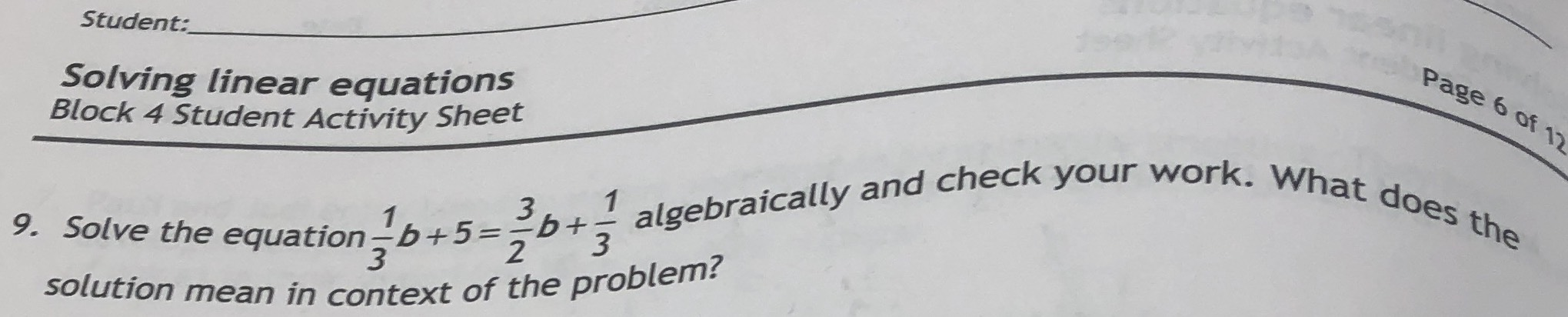### ¿Todavía tienes preguntas de matemáticas?

Pregunte a nuestros tutores expertos
Algebra
Pregunta9. Solve the equation $$\frac { 1 } { 3 } b + 5 = \frac { 3 } { 2 } b + \frac { 1 } { 3 }$$ algebraically and check your work. What does the solution mean in context of the problem?